##### Geometry For Dummies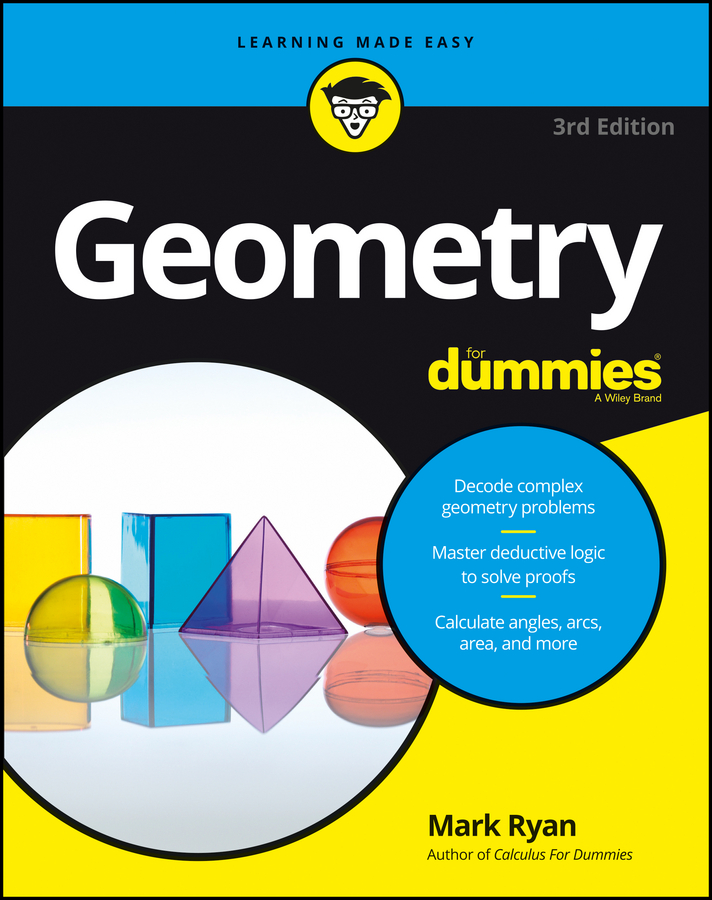The AAS (Angle-Angle-Side) theorem states that if two angles and a nonincluded side of one triangle are congruent to the corresponding parts of another triangle, then the triangles are congruent. The following figure shows you how AAS works.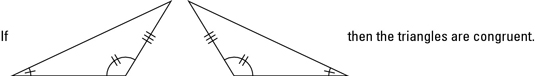Like ASA (angle-side-angle), to use AAS, you need two pairs of congruent angles and one pair of congruent sides to prove two triangles congruent. But for AAS, the two angles and one side in each triangle must go in the order angle-angle-side (going around the triangle either clockwise or counterclockwise). Another way to look at it is that if you’ve got two angles and a side and you don’t have ASA, it’s got to be AAS.

ASS (angle-side-side) and SSA (side-side-angle) don’t prove anything, so don’t try using ASS (or its backward twin, SSA) to prove triangles congruent. You can use SSS (side-side-side), SAS (side-angle-side), ASA (angle-side-angle), and AAS (or SAA, the backward twin of AAS) to prove triangles congruent, but not ASS. In short, every three-letter combination of A’s and S’s proves something unless it spells ass or is ass backward. (By the way, AAA proves triangles similar, not congruent.)

Try to solve the following proof by first looking for all isosceles triangles (with the two isosceles triangle theorems in mind) and for all pairs of congruent triangles (with CPCTC — Corresponding Parts of Congruent Triangles are Congruent — in mind).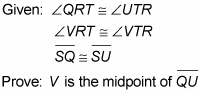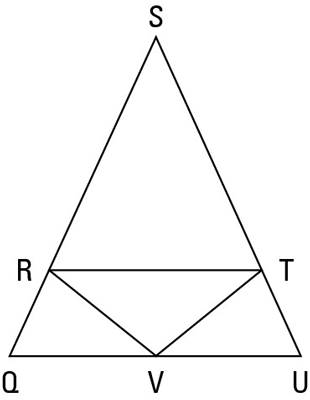Here’s a game plan that shows how you might think through this proof:

• Take note of isosceles triangles and pairs of congruent triangles.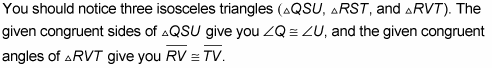You should also notice the two congruent-looking triangles (triangle QRV and triangle UTV) and then realize that showing them congruent and using CPCTC is very likely the ticket.

• Look at the prove statement and work backwards. To prove the midpoint, you need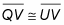on the second-to-last line, and you could get that by CPCTC if you knew that triangle QRV and triangle UTV were congruent.

• Figure out how to prove the triangles congruent. You already have (from the first bullet) a pair of congruent angles (angle Q and angle U) and a pair of congruent sides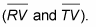Because of where these angles and sides are, SAS and ASA won’t work, so the key has to be AAS. To use AAS, you’d need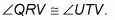Can you get that? Sure. Check out the givens: You subtract congruent angles VRT and VTR from congruent angles QRT and UTR. Checkmate.

Here’s the formal proof:

Statement 1: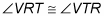Reason for statement 1: Given.

Statement 2: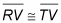Reason for statement 2: If angles, then sides.

Statement 3: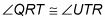Reason for statement 3: Given.

Statement 4:

Reason for statement 4: If two congruent angles (angle VRT and angle VTR) are subtracted from two other congruent angles (angle QRT and angle UTR), then the differences (angle QRV and angle UTV) are congruent.

Statement 5: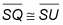Reason for statement 5: Given.

Statement 6: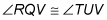Reason for statement 6: If sides, then angles.

Statement 7: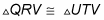Reason for statement 7: AAS (using lines 6, 4, and 2).

Statement 8:Reason for statement 8: CPCTC.

Statement 9: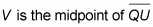Reason for statement 9: Definition of midpoint.